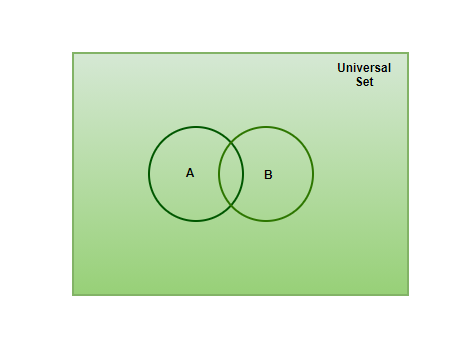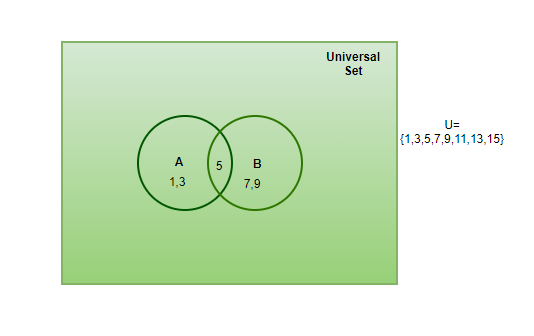# Subsets, Power Sets and Universal Sets

• Last Updated : 26 May, 2021

In order to understand things properly and to remember them for a long time, human beings prefer to keep information in bunches or categories, the same type of information goes in one category, this way, information looks neater and becomes easier to remember. Sets are also defined in the same way. In order to keep elements belonging to the same category, they are kept in one set, this way in mathematics, data can be neatly arranged as well. However, sets do not allow the mentioning of the qualities of elements. For Instance, all the girls in class 8 can be put in one set but all the pretty girls in class 8 can not be considered an example of a set. Let’s learn about sets in more detail.

### Sets

Sets are defined as the well-defined collection of data. The data present in the set is known as an element, each element belonging to one set are different from the other but the category they belong to is always the same, for instance, a bag of different types of fruits, all-natural numbers, etc.

Attention reader! All those who say programming isn't for kids, just haven't met the right mentors yet. Join the  Demo Class for First Step to Coding Coursespecifically designed for students of class 8 to 12.

The students will get to learn more about the world of programming in these free classes which will definitely help them in making a wise career choice in the future.

Examples of Sets:

• {1, 2, 3, 4, 5} ⇢ Example of Finite set.
• {} ⇢ Example of Null Set.
• {a: a is an odd number} ⇢ Example of Infinite set.
• {p} ⇢ Example of singleton set.

### Subsets

If a Set has all its elements belonging to other sets, this set will be known as a subset of the other set. A Subset is denoted as “⊆ “. If set A is a subset of set B, it is represented as A⊆ B.

Example of a Subset,

Set A= {m, n, o, p, q}

Set B= {k, l, m, n, o, p, q, r}

Then, A ⊆ B

Proper Set

If set A has all its elements present in set B and set B has more number of elements then set A is a proper set of set B. The proper set is represented as ‘⊂’

Two sets having an equal number of elements can never be proper sets of each other, hence, a set is never a proper set of itself.

Note: All Proper sets are Subsets, but all Subsets can not be considered as Proper sets.

### Power Sets

Power Set is defined as the set of all the possible subsets of the given set. The definition seems a little confusing but in real, Power sets are very easily understood. Imagine a set with some elements present in them, now write all the possible subsets that can be written for the particular set, treat the subsets as elements as place them in a separate set, this set obtained will be called as a Power set.

Example of a Power set,

Set A = {2,3}

Possible Subsets of A = {}, {2}, {3}, {2, 3}

Power set of A = P(A)= {{}, {2}, {3}, {2, 3}}

Note: If there are ‘n’ number of elements, then the number of elements present in the Power set will be 2n.

### Universal Set

Universal set is the master of all the sets, that is, it contains all the elements present in all the sets given. The universal set is represented as U, and it is represented as a rectangle in the Venn diagram, all the other sets are drawn within the rectangle, this is done to show that the universal set contains all the possible elements.

Assume set A and set B are parts of the universal sets,

Set A = {1, 2, 3, 4}

Set B= {2, 4, 5, 6}

U= {1, 2, 3, 4, 5, 6}

This should look something like this on the Venn diagram,### Sample Problems

Question 1: State if the following statements are True or False,

1. Every Set is a subset of itself
2. If all the elements of Set A are present in Set B, Set B becomes the subset of Set A.
3. A Universal is never a subset of any other set.
4. Not all sets are subsets of the Universal set.
5. A Power Set does not include an empty set.

1. True. Every set can be denoted as a subset of itself.
2. False. The true statement should be that set A ⊂ set B.
3. True. Universal set contains all the elements in itself.
4. False. All the sets are subsets of U.
5. False. A Power set includes empty set.

Question 2: Explain which of the following sets are the subsets of Set P,

Set P = {1, 3, 5, 7, 9, 11, 13, 15,17,19}

1. Set A = {a, 1, 0, 2}
2. Set B ={3, 7, 9}
3. Set C = {1, 4, 6, 11}
4. Set D = {19,1}
5. Set E ={9, 13, 15, 17}

1. Set A has elements a, 1, 0, 2 which are not present in the Set P. Therefore, set A is not a Subset.
2. Set B has elements which are present in set P, Therefore, Set B ⊆ Set P
3. Set C has 2 extra elements. Hence, not a subset of P
4. Set D has 1, 19 as element. Therefore, Set D ⊆ Set P
5. Set E has all its elements matching the elements of set P. Hence, Set E ⊆ Set P.

Question 3: What type of elements can not be put in a Set?

Anything that talks about quality or characteristic of the elements, like the good, the bad, can not be put in a set. For example, “all the good football players “This statement can not be put in a set, Or” all the students who performed poor in the class” can not be put in a set.

Question 4: What is the difference between a Subset and a Proper set?

If a set A has all its elements present in set B, then it can be said that set A ⊆ set B, one important thing to notice is that both sets can be equal and still be subsets of each other.

If set A has all its elements present in set B and the elements in set B are more than the elements in set A, then it can be said that set A is a proper set of set B. It is represented as A B.

Question 5: What is the Power Set of Set P= {a, b, c}?

There are 3 elements in the set P, therefore, it will have 23 elements present in its Powerset. It can also be said that there are 8 subsets possible for set P.

Subsets of P= {}, {a}, {b}, {c}, {a, b}, {b, c}, {a, c}, {a, b, c}.

Power set of P= {{}, {a}, {b}, {c}, {a, b}, {a, c}, {b, c}, {a, b, c}}.

Question 6: Represent the information given below in Venn diagram,

U= {1, 3, 5, 6, 7, 9,11, 13, 15}

Set A= {1, 3,5}

Set B= {5, 7, 9}